Try out our new practice tests completely free!# Quiz 29: Magnetic Fields Due to CurrentsTwo long straight wires pierce the plane of the paper at vertices of an equilateral triangle as shown below.They each carry 2.0 A, out of the paper.The magnetic field at the third vertex (P)has magnitude: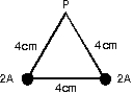(Multiple Choice)

DSuitable units for 0 are:(Multiple Choice)

ETwo long straight wires are parallel and carry current in the same direction.The currents are 8.0 and 12 A and the wires are separated by 0.40 cm.The magnetic field at a point midway between the wires is:(Multiple Choice)

BElectrons are going around a circle in a counterclockwise direction as shown.At the center of the circle they produce a magnetic field that is: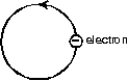(Multiple Choice)In the figure, if the current element has a length of 1.0 mm, carries a current of 2.5 A, and is a distance of 4.8 cm from the point P, what is the magnitude of the magnetic field at point P?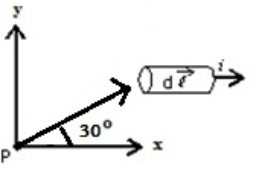(Multiple Choice)In an overhead straight wire, the current is north.The magnetic field due to this current, at our point of observation, is:
(Multiple Choice)The diagrams show three circuits consisting of concentric circular arcs (either half or quarter circles of radii r, 2r, and 3r)and radial lengths.The circuits carry the same current.Rank them according to the magnitudes of the magnetic fields they produce at C, least to greatest.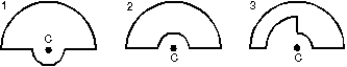(Multiple Choice)The magnetic field a distance 2 cm from a long straight current-carrying wire is 2 10-5 T.The current in the wire is:
(Multiple Choice)Two long straight wires are parallel and carry current in opposite directions.The currents are 8.0 A and 12 A and the wires are separated by 0.40 cm.The magnetic field at a point midway between the wires is:
(Multiple Choice)Two long straight current-carrying parallel wires cross the x axis and carry currents I and 3I in the same direction, as shown.At what value of x is the net magnetic field zero?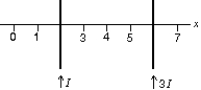(Multiple Choice)An ordinary magnetic compass is placed flat on the ground.A wire carrying a large current i from east to west is placed directly above the compass.The end of the compass needle marked "N" will point:
(Multiple Choice)Two long parallel straight wires carry equal currents in opposite directions.At a point midway between the wires, the magnetic field they produce is:
(Multiple Choice)The magnetic field outside a long straight current-carrying wire depends on the distance R from the wire axis according to:
(Multiple Choice)Which graph correctly gives the magnitude of the magnetic field outside an infinitely long, very thin, straight current-carrying wire as a function of the distance r from the wire?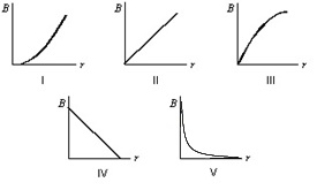(Multiple Choice)A coulomb is:
(Multiple Choice)In the figure, the current element , the point P, and the three vectors (1, 2, 3)are all in the plane of the page.The direction of , due to this current element, at the point P is: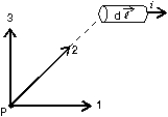(Multiple Choice)Lines of the magnetic field produced by a long straight wire carrying a current are:
(Multiple Choice)The magnitude of the magnetic field at point P, at the center of the semicircle shown, is given by: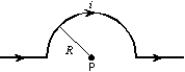(Multiple Choice)A semi-infinite wire runs along the positive y axis, beginning at the origin (x = y = 0)and extending infinitely far in the +y direction.If the current in the wire is 12 A, what magnetic field does it create on the x axis at the point x = 3.5 cm?
(Multiple Choice)The diagram shows three equally spaced wires that are perpendicular to the page.The currents are all equal, two being out of the page and one being into the page.Rank the wires according to the magnitudes of the magnetic forces on them, from least to greatest.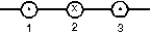(Multiple Choice)## Filters

• Essay(0)
• Multiple Choice(0)